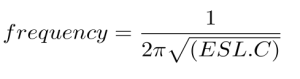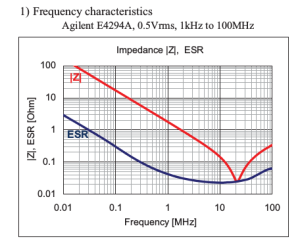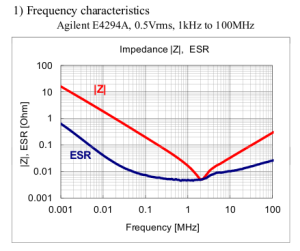# Calculating ESL of a Capacitor

Impedance of Various 100μF Capacitors :• The figure tells us that the impedance of a capacitor will decrease monotonically as frequency is increased.

• In actual practice, the ESR causes the impedance plot to flatten out.

• As we continue up in frequency, the impedance will start to rise due to the ESL of the capacitor.

• The location and width of the “knee” will vary with capacitor construction, dielectric and value.

• This is why we often see larger value capacitors paralleled with smaller values. The smaller value capacitor will typically have lower ESL and continue to “look” like a capacitor higher in frequency.

• This extends the overall performance of the parallel combination over a wider frequency range.Reference : From Analog Devices Tutorial

Frequency Characteristics of a 0.1 uf Capacitor :The impedance matches with ESR at around at around 2 Mhz.

ESL Analysis :

Frequency = 20 Mhz

Capacitance = 0.1 uF

From the frequency equation , ESL = 0.63 nH

Frequency Characteristics of a 1 uf Capacitor :

The impedance matches with ESR at around at around 8 Mhz.

ESL Analysis :

The impedance matches with ESR at around at around 8 Mhz.

Frequency = 8 Mhz

Capacitance = 1 uF

From the frequency equation , ESL = 0.39 nH

The impedance matches with ESR at around at around 2 Mhz.

ESL Analysis :

Frequency = 2 Mhz

Capacitance = 10 uF

From the frequency equation , ESL = 0.63 nH

Frequency Characteristics of a 10uf Capacitor :The impedance matches with ESR at around at around 2 Mhz.

ESL Analysis :

Frequency = 2 Mhz

Capacitance = 10 uF

From the frequency equation , ESL = 0.63 nH

Capacitor Graph Reference : Datasheets from Digikey